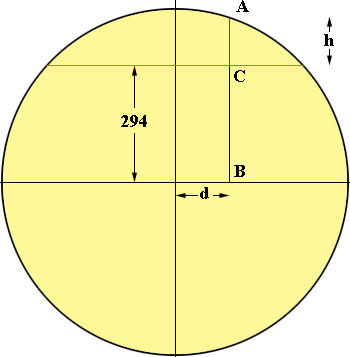SEARCH HOMEMath Central Quandaries & QueriesHere is my question. Imagine I have a circle of known radius 25 feet, and a chord with a mid point height of 6 inches from a central point on the chord to the circumference of the circle. I wish to divide this chord into a number of equal divisions. How can I calculate the measurement of the perpendicular line at each division of the chord to the circumference and at a 90 degrees at each divisionAustin,

The equation of a circle with its centre at the origin is x2 + y2 = r2 where r is the radius. For your problem I think it will be more convenient to work in inches rather than feet so the equation of the circle is

x2 + y2 = (25 × 12)2 = 3002 = 90,000Suppose you move d inches away from the midpoint of the chord. You want the height h in the diagram. Since the equation of the circle is

x2 + y2 = 90,000

we have

d2 + y2 = 90,000

where y is the length of the line segment AB. Since the length of BC is 300 = 6 = 294 inches

d2 + (294 + h)2 = 90,000
(294 + h)2 = 90,000 - d2

so

h = √(90,000 - d2) - 294 inches.

Thus, for example if d = 1 ft = 12 inches then

h = √(90,000 - 122) - 294 = √(90,000 - 144) - 294 = √(89856) - 294 = 299.76 - 294 = 5.76 inches

I hope this helps,
PennyMath Central is supported by the University of Regina and The Pacific Institute for the Mathematical Sciences.